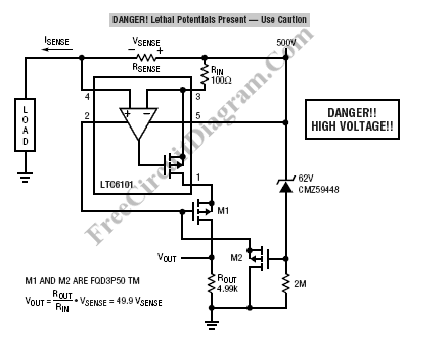# Current Sensing for High Voltage ApplicationHigh voltage current sensing/monitoring can be designed using LTC6101 op-amp. The following current sensing circuit is capable of measuring electrical current in 500 volts system. Here is the schematic diagram of the circuit:Two external Mosfets is added to hold off the voltage, allows the LTC6101 to be connected to a very high potentials and measure the current flow. The output current from the op-amp, that is proportional to the sensed voltage at Rsense, flows through M1 and create a ground referenced output voltage. [Schematic diagram source: Linear Technology Application Notes]